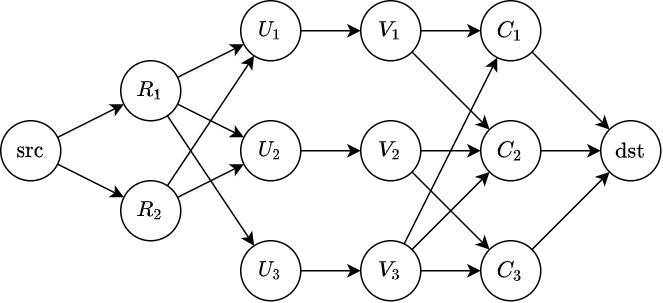Contest Duration: - (local time) (100 minutes) Back to Home

## F - Grid and Tokens Editorial by en_translator

Rephrase the problem as follows:

Given are foods $$R_1, R_2, \dots, R_H$$ and drinks $$C_1, C_2, \dots, C_W$$.

Each of $$N$$ people has a preference of foods and drinks. The preference of person $$i \, (1 \leq i \leq N)$$ is:

• The person wants one of $$R_{a_i}, R_{a_i + 1}, \dots, R_{c_i}$$, and one of $$C_{b_i}, C_{b_i + 1}, \dots, C_{d_i}$$.

Supposing that the same drink or food cannot be assigned to multiple people, what is the maximum number of people whose preferences are satisfied?

This can be boiled down to a maximum flow problem.

• Prepare the following vertex.
• $$\mathrm{src}, \mathrm{dst}$$
• $$R_1, \dots, R_H$$
• $$C_1, \dots, C_W$$
• $$U_1, \dots, U_N$$
• $$V_1, \dots, V_N$$
• Add an edge with capacity $$1$$ from $$\mathrm{src}$$ to every $$R_i$$.
• For each $$i \, (1 \leq i \leq N)$$,
• add an edge with capacity $$1$$ from $$R_{a_i}, R_{a_i + 1}, \dots, R_{c_i}$$ to $$U_i$$.
• add an edge with capacity $$1$$ from $$U_i$$ to $$V_i$$.
• add an edge with capacity $$1$$ from $$V_i$$ to $$C_{b_i}, C_{b_i + 1}, \dots, C_{d_i}$$.
• Add an edge with capacity $$1$$ from every $$C_i$$ to $$\mathrm{dst}$$.
• Compute the maximum flow from $$\mathrm{src}$$ to $$\mathrm{dst}$$.

The graph corresponding to the Sample Input 1 is shown below.If we use the Ford-Fulkerson algorithm, it can be solved in a total of $$O(FE)$$ time; if Dinic’s algorithm is used, it can be solved in a total of $$O(E\sqrt V)$$, where $$V = H + W + N, E = (H + W)N, F = \min(H, W, N)$$.

Sample code (C++)

posted:
last update: